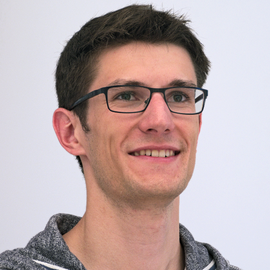# Image Classification Using Wavelet und Shearlet Based Scattering Transforms

Contributed Talk

### Abstract

The goal of statistical learning is to fit a prediction model to a set of training data that were drawn i.i.d. according to an unknown joint probability distribution on the space of inputs and outputs. The trained model is then used to estimate the conditional probability distribution on the space of outputs given some previously unseen input data.

A major question is how well such a trained model generalizes to new data that was not used during the training. Or in other words, how well can we control the error that was made by approximating the underlying unknown distribution by using only a finite sample of training data. And how does this error behave when we increase the number of samples?

The answers to these questions depend heavily on the capacity of the prediction model. In many cases predictions can be improved by exploiting certain invariants of the data, e.g. in image classification tasks an invariance with respect to translations or small diffeomorphic deformations. This motivated the incorporation of wavelet based Scattering Transforms (S. Mallat, 2012) into prediction models.

Here we consider the case of a multi-class classification problem with multinomial logistic regression classifier model and wavelet or shearlet based Scattering Transform feature extractor and derive a bound on the generalization error using covering numbers.

As an example we study the problem of digit recognition and present results for the MNIST database of handwritten digits.

Date
Feb 3, 2018 9:00 AM — 9:30 AM
Location
Bergkloster Bestwig
Bestwig,##### Jan Macdonald

My research is at the interface of applied and computational mathematics and scientific machine learning. I am interested in inverse problems, signal- and image recovery, and robust and interpretable deep learning.